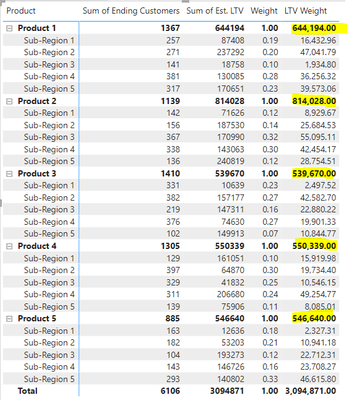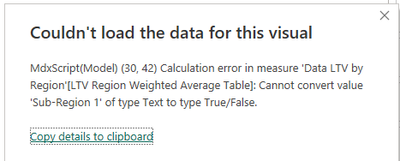cancel
Showing results for
Did you mean:New Member

## Weighted Average with ALLSELECTED()

Hi,

I'm struggling to compute a weighted average with my dataset. I'm looking to calculate a weighted average for product by sub-region. I also want the calculation to respond to filters applied on the sub-region column (if I take some regions out I still want the weight % to sum to 1). Sample dataset here:Matrix visual here: I've got the weight percentage to work correctly and used ALLSELECTED() to have the weight respond correctly to any filters applied on the Sub-Region field. However I'm struggling to get the Product subtotal to sum the values below it, instead it just takes the Sum from 2nd value column.See code below for weight:

``````Weight =
DIVIDE(
SUM('Data LTV by Region'[Ending Customers]),
CALCULATE(SUM('Data LTV by Region'[Ending Customers]), ALLSELECTED('Data LTV by Region'[Sub-Region])
)
)``````

Code for the weighted LTV

``````LTV Weight =
[Weight]*sum('Data LTV by Region'[Est. LTV])``````

Simpler attempt at getting the weighted average at the Product subtotal

``````Product Weighted =
IF(
ISINSCOPE('Data LTV by Region'[Product]),
SUMX('Data LTV by Region', [LTV Weight]),
SUM('Data LTV by Region'[Est. LTV])
)``````

Tried using SUMMARIZE and writing something a little more complex to return the sum of a weighted average column but admittedly not too experienced with using SUMMARIZE. Code below and underneath is the error I'm getting.

``````LTV Region Weighted Average Table =
VAR CustomersEnding =
SUM('Data LTV by Region'[Ending Customers])
VAR LTV =
SUM('Data LTV by Region'[Est. LTV])
VAR CustomersEndingTotal =
CALCULATE(CustomersEnding, REMOVEFILTERS('Data LTV by Region'[Sub-Region]))
VAR Wgt =
CALCULATE(DIVIDE(CustomersEnding, CustomersEndingTotal))
VAR WeightedAvgTable =
SUMMARIZE(
FILTER('Data LTV by Region', ALLSELECTED('Data LTV by Region'[Sub-Region])),
'Data LTV by Region'[Product], 'Data LTV by Region'[Sub-Region]
),
"Weight", Wgt,
"LTV", LTV
)
RETURN
SUMX(WeightedAvgTable,
[Weight]*[LTV]
)``````My end goal would be to put this into a SWITCH() statement since I've figured out all the other parts of the formula and how to get it to work in the matrix visual. This piece has been trickier than I anticipated but feel like I'm inexperienced and missing something simple. Any help or suggestions would be appreciated with figuring this out.

Thanks!Super User

LTV Region Weighted Average Table =
VAR CustomersEnding =
SUM('Data LTV by Region'[Ending Customers])
VAR LTV =
SUM('Data LTV by Region'[Est. LTV])
VAR CustomersEndingTotal =
CALCULATE(CustomersEnding, REMOVEFILTERS('Data LTV by Region'[Sub-Region]))
VAR Wgt =
CALCULATE(DIVIDE(CustomersEnding, CustomersEndingTotal))
VAR WeightedAvgTable =
SUMMARIZE(
calculateTable('Data LTV by Region',, ALLSELECTED('Data LTV by Region'[Sub-Region]))
'Data LTV by Region'[Product], 'Data LTV by Region'[Sub-Region]
),
"Weight", Wgt,
"LTV", LTV
)
RETURN
SUMX(WeightedAvgTable,
[Weight]*[LTV]
)

!! Microsoft Fabric !!
Microsoft Power BI Learning Resources, 2023 !!
Learn Power BI - Full Course with Dec-2022, with Window, Index, Offset, 100+ Topics !!
Did I answer your question? Mark my post as a solution! Appreciate your Kudos !! Proud to be a Super User! !!